# Electronics and Communication Engineering - Networks Analysis and Synthesis

41.

The time constant of the circuit in figure shown.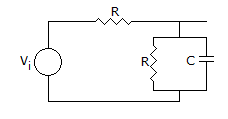A. RC B.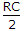C.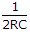D.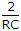Explanation:

Time constantReq.C

when V is shorted and R is seen from C then Req = R || R = R/2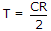.

42.

In the circuit shown in given figure, the values of i(0+) and I(∞), will be respectively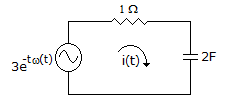A. zero and 1.5 A B. 1.5 A and 3 A C. 3 A and zero D. 3 A and 1.5 A

Explanation: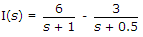I(t) = 6 e-t - 3 e-0.5t

at t = 0, I(0) = 3A

at t = ∞, I(∞) = 0.

43.

A two branch parallel circuit has a 20 Ω resistance and 1 H inductance in one branch and a 100 μF capacitor in the second branch. It is fed from 100 V ac supply, at resonance, the input impedance of the circuit is

 A. 500 Ω B. 50 Ω C. 20 Ω D. 5 Ω

Explanation:

At parallel resonance circuit impedance =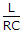.

44.

The impedance matrices of two, two-port network are given by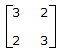and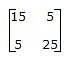. If these two networks are connected in series, the impedance matrix of the resulting two-port network will be

 A.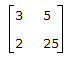B.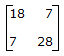C.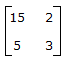D. indeterminate

Explanation:

45.

As compared to the number of network elements in Brune synthesis, the number of network elements Bott-and Duffin in realization is

 A. more B. less C. equal D. any of the above